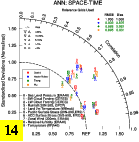f.e20.F2000.f09_f09.195.007_pia
and
f.e20.F2000.f09_f09.195.007

DIAG Set 14 - Taylor Diagrams

Taylor Diagrams were developed by Karl Taylor at PCMDI (paper|tech note) and aim to condense information about variance and RMSE characteristics of a particular model run when compared with observations in a single diagram. The tables summarize the individual metrics for each variable considered including:

• bias, as absolute percentage difference from observations
• variance, as a ratio of the observed variance
• correlation, correlation coefficient with observations

## Taylor Diagrams

Space and time ANN
Space only ANN DJF MAM JJA SON

## Bias(%), Variance (ratio), Correlation Coefficient Tables

Space and time correlation variance bias
Space only correlation variancebias
Time only correlation